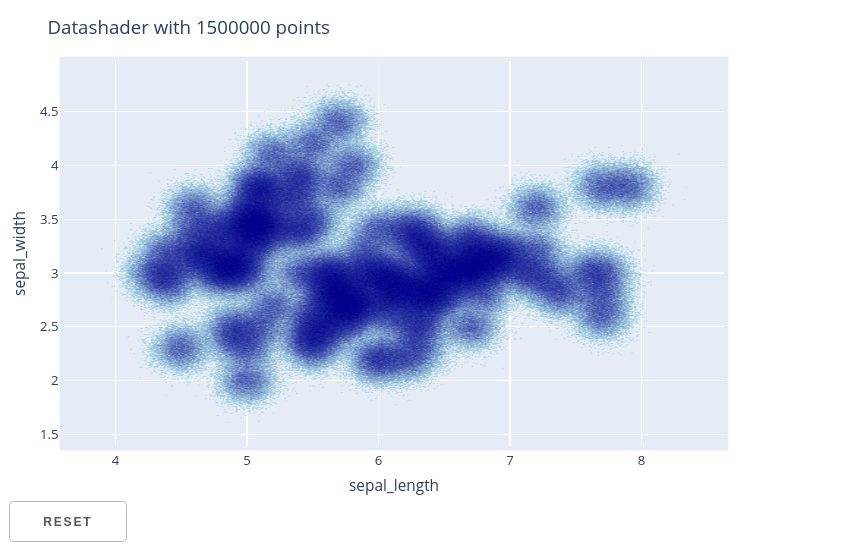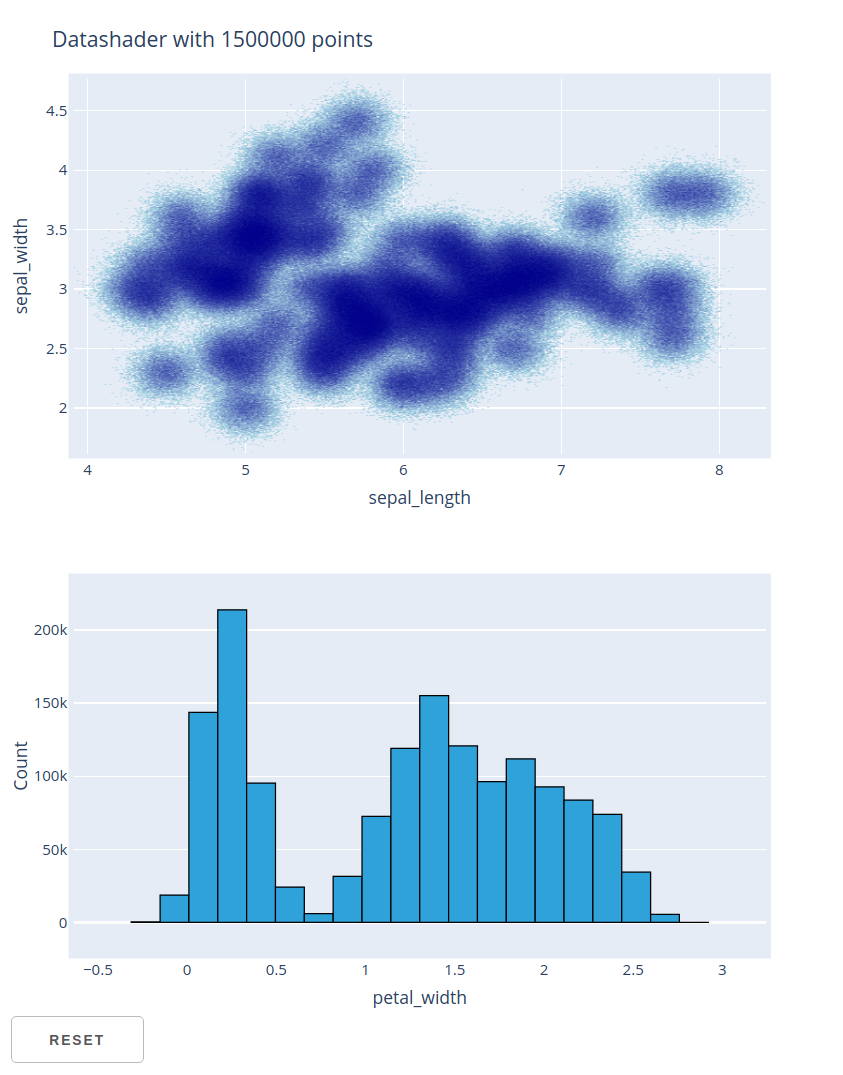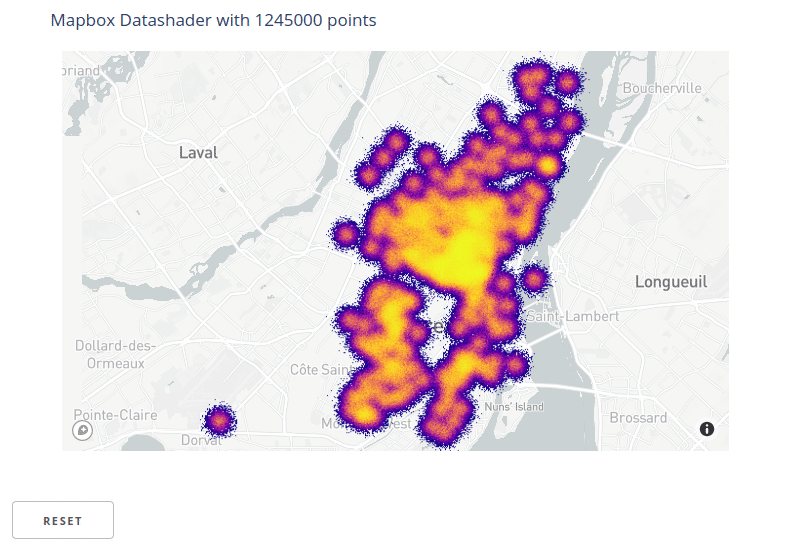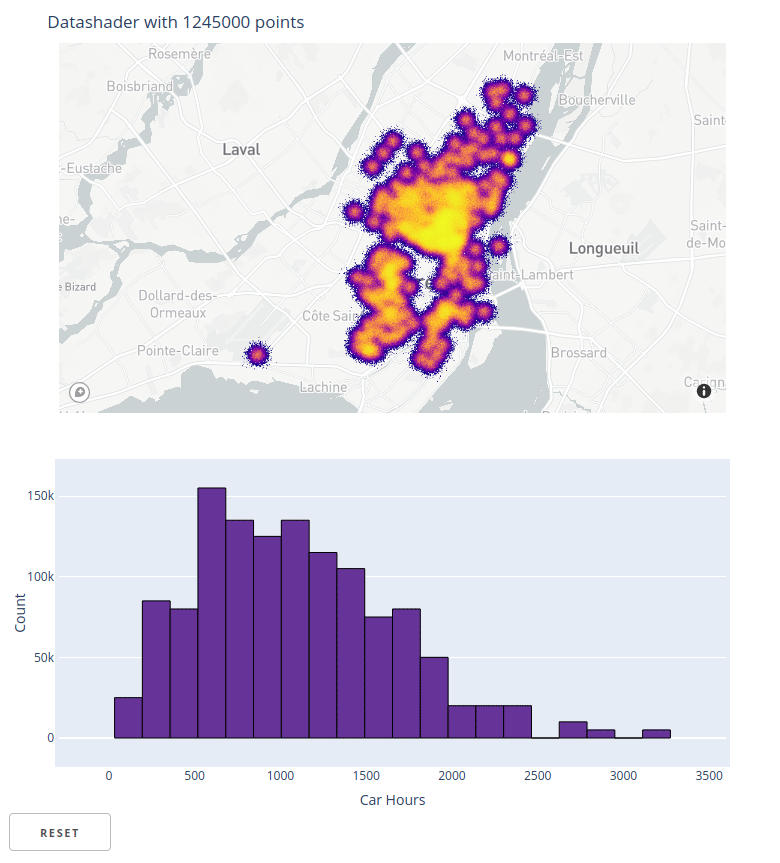#HoloViews Overview

HoloViews is an ambitious project that aims to provide a flexible grammar of
visualization types and plot interactions. HoloViews specifications can be
displayed using a variety of technologies, including Plotly.js and Dash.

While HoloViews can be used to create a large variety of visualizations, for Dash
users it is particularly helpful for two use cases: Automatically linking selections
across multiple figures and displaying large data sets using Datashader.

HoloViews also provides a uniform interface to a variety of data structures,
making it easy to start out by visualizing small pandas DataFrames and then scale
up to GPU accelerated RAPIDS cudf DataFrames, or larger than memory Dask DataFrames.

For more background information, see the main HoloViews documentation at
https://holoviews.org/

## HoloViews Elements and Containers

The visualization primitives in HoloViews are called elements. Elements
in HoloViews are analogous to Plotly traces, and there are specific elements for
`Scatter`,
`Area`,
`Bars`,
`Histogram`,
`Heatmap`,
etc.

Elements can be grouped together into various containers including the
`Overlay`
container for overlaying multiple compatible elements on the same axes, and the
`Layout`
container for displaying multiple elements side by side as separate subplots.
to aid in the visualization of multi-dimensional datasets including the
`HoloMap`,
`Gridspace`,
`NdLayout`,
and
`Ndoverlay`
containers.

Finally, the
`DynamicMap`
is a special container than produces elements dynamically, often
in response to user interaction events. This documentation page does not discuss
the creation of general `DynamicMap` instances, but it’s helpful to understand
that the `datashade` and `link_selections` transformations discussed below both
produce either `DynamicMap` instances, or containers of `DynamicMap` instances.

## HoloViews Datasets

While it’s possible to build HoloViews elements directly from external data
structures like numpy arrays and pandas DataFrames, HoloViews also provides a
`Dataset` class that aims to serve as a universal interface to these data
structures. The recommended workflow is to first wrap the original data structure
(e.g. the pandas DataFrame) in a `Dataset` instance, and then construct
elements using this `Dataset`.

This workflow has two main advantages:

1. It makes it easy to swap out data structures in the future. For example, you
could develop a visualization using a small pandas DataFrame and then later
switch to a GPU accelerated cuDF DataFrame or a larger-than-memory dask
DataFrame.

2. It allows HoloViews to associate each visualization element with all of the
dimensions (i.e. columns in the case of a DataFrame) in the original `Dataset`.
This is what makes it possible for HoloViews to automate the process of
linking selections across visualizations that do not all display the same
dimensions. See the following sections for some examples of using the
`link_selections` function to accomplish this.

The examples in this documentation page use `Dataset` instances that wrap tabular
data structures. But Datasets also support wrapping gridded datasets like numpy
`ndarray`
and xarray
`DataArray`
objects. See the
Tabular Datasets
see the
Gridded Datasets

## Building Dash Components from HoloViews Objects

HoloViews elements and containers can be converted into Dash components using the
`holoviews.plotting.plotly.dash.to_dash` function. This function inputs
a `dash.Dash` app instance and a list of HoloViews objects, and returns a
`namedtuple` with the following properties.

• `graphs`: This is a list of converted `Graph` components with the same
length as the input list of HoloViews objects. By default, these will have
type `dash_core_components.Graph`, but an alternative graph component class
(e.g. `dash_design_kit.Graph`) can be specified using the `graph_class`
argument to the `to_dash` function.
• `resets`: If the `reset_button=True` argument is passed to
`to_dash`, the `resets` property will hold a length 1 list
containing a Dash component that represents a reset button. When clicked,
this button will reset the graphs to their initial state. This will reset
both the figure viewports and other interactive states like the active
selection produced by the `link_selection` examples below. If
`reset_button=False`, the default, then this list will be empty.
• `store`: A Dash `Store` component that is used
internally to maintain the joint interactive state of all of the converted
Dash components.
• `kdims`: For Dimensioned HoloViews containers, the `kdims`
property holds a dictionary from key-dimension names to Dash components
that represent sliders for each key dimension. Dimensioned Containers are
not discussed further here; see the
Dimensioned Containers
• `children`: This is a list that contains all of the components above. This can
be assigned directly to the `children` property of an `html.Div` component if
no additional layout structure is needed.

After calling `to_dash`, each of the resulting components must be
included somewhere in the app’s layout. This can be done by either:

• including the `children` list as the `children` property of an `html.Div`
component.
• including all of the components stored in `graphs`, `resets`, `kdims`, and
`store` somewhere in the app’s layout.

## Display Simple HoloViews Elements with Dash

This example loads the iris dataset included in plotly.py and wraps it in a
HoloViews `Dataset`. This `Dataset` is then used to construct a
`Scatter`
element and a
`Histogram`
element. The `Histogram` element is created using the
`histogram`
operation which is what executes the histogram binning algorithm.

These two elements are converted into two Dash `Graph` components using the
`to_dash` function, and are placed into a `Div` component along with the
associated `Store` component.

``````from dash import Dash, html
from plotly.data import iris

import holoviews as hv
from holoviews.plotting.plotly.dash import to_dash

df = iris()
dataset = hv.Dataset(df)

scatter = hv.Scatter(dataset, kdims=["sepal_length"], vdims=["sepal_width"])
hist = hv.operation.histogram(
dataset, dimension="petal_width", normed=False
)

app = Dash(__name__)
components = to_dash(app, [scatter, hist])

app.layout = html.Div(components.children)

if __name__ == "__main__":
app.run(debug=True)
``````

## Styling Figures Produced by HoloViews

There are two general approaches that can be used to customize the appearance
of the Plotly figures produced by HoloViews.

### Options System

The first is the HoloViews options system. This approach uses the syntax:

``````element.opts(option1=value1, option2=value2)
``````

This is very analogous to the `fig.update()` syntax that is used to update Plotly
`plotly.graph_object.Figure` objects. The available options for a particular
element type can be discovered from a Python or IPython REPL using the
`holoviews.help` function. For example:

``````import holoviews as hv
hv.extension("plotly")
hv.help(hv.Scatter)
``````

A slightly different syntax is used to style elements inside a container. Here is
an example of how `Scatter` element options would be applied to a container that
contains or produces `Scatter` elements:

``````from holoviews import opts
container.opts(opts.Scatter(option1=value1, option2=value2))
``````

This makes it possible to target options to elements of specific types within
a container. This is the approach that must be used to apply options to the
`DynamicMap` instances produced by the `datashade` and `link_selections`
transformations discussed below.

The example below customizes the appearance of a `Scatter` element using the
`size` and `color` options.

See the
Applying Customizations
usng the options system.

### Plot Hooks

HoloViews aims to expose the most common plot options through the `opts` syntax
above, but the coverage of possible plotly configuration options is not exhaustive.
HoloViews provides a system called “plot hooks” to make it possible to apply arbitrary
figure customizations. Every element has a special option named `hooks` that may
be set to a list of functions that should be applied to the figure that HoloViews
generates before it is displayed.

The example below uses a plot hook to change the default drag tool from
`zoom` to `pan`.

See the
Plot hooks

``````from dash import Dash, html
from plotly.data import iris

import holoviews as hv
from holoviews.plotting.plotly.dash import to_dash

df = iris()
dataset = hv.Dataset(df)

scatter = hv.Scatter(dataset, kdims=["sepal_length"], vdims=["sepal_width"])

# Set scatter size and color using options system
scatter.opts(size=15, color="purple")

# Set default drag mode to pan using a plot hook
def hook(plot, element):
fig = plot.state
fig["layout"]["dragmode"] = "pan"
scatter.opts(hooks=[hook])

app = Dash(__name__)
components = to_dash(app, [scatter])

app.layout = html.Div(components.children)

if __name__ == "__main__":
app.run(debug=True)
``````

One HoloViews feature that is particularly convenient for Dash users is the ability
to automatically link selections across figures without the need to manually define
any callback functions.

This can be done by first creating a `link_selections` instance
(called `selection_linker` in the examples below) using the
`link_selections.instance()` method, and then calling this object as a function
with the elements or containers to be linked.

When these linked elements are passed to the `to_dash` function, Dash
callbacks to achieve this interactive linking behavior are automatically generated
and registered with the provided `dash.Dash` app instance.

This example shows how the `reset_button=True` argument to `to_dash` can
be used to create a Dash button component. When this button is clicked, the plot
viewport and selection states are reset to their original values.

For more background on linked selections in Holoviews, see the
documentation section.

Try using the box-selection tool to select regions of space in each figure and
notice how the selection of the corresponding data is displayed in both figures.
Note that only box selection is supported right now. Lasso selection support
is not yet implemented.

``````from dash import Dash, html
from plotly.data import iris

import holoviews as hv
from holoviews import opts
from holoviews.plotting.plotly.dash import to_dash

df = iris()
dataset = hv.Dataset(df)

hv.Scatter(dataset, kdims=["sepal_length"], vdims=["sepal_width"])
)
hv.operation.histogram(dataset, dimension="petal_width", normed=False)
)

# Use plot hook to set the default drag mode to box selection
def set_dragmode(plot, element):
fig = plot.state
fig['layout']['dragmode'] = "select"
if isinstance(element, hv.Histogram):
# Constrain histogram selection direction to horizontal
fig['layout']['selectdirection'] = "h"

scatter.opts(opts.Scatter(hooks=[set_dragmode]))
hist.opts(opts.Histogram(hooks=[set_dragmode]))

app = Dash(__name__)
components = to_dash(
app, [scatter, hist], reset_button=True
)
app.layout = html.Div(components.children)

if __name__ == "__main__":
app.run(debug=True)
``````

## Visualizing Large Datasets with Datashader

Another HoloViews feature that is particularly convenient for Dash users is the

Datashader is a Python library for quickly creating a variety of principled
visualizations of large datasets.

While the Plotly `scattergl` trace can handle hundreds of thousands of points,
Datashader can handle tens to hundreds of millions. The difference is that rather
than passing the entire dataset from the Python server to the browser for rendering,
Datashader rasterizes the dataset to a heatmap or image, and only this heatmap or
image is transferred to the browser for rendering.

To effectively use Datashader in an interactive context, it’s necessary to rerender
the dataset each time the figure viewport changes. This can be accomplished in
Dash by installing a callback function that listens for changes to the
`relayoutData` prop. Because of how HoloViews packages data lazily (without
rendering it immediately), replaying this pipeline of transformations can be
accomplished without manually defining any callbacks, making Datashader much easier
to use than if invoked without HoloViews.

This example loads the iris dataset included in plotly.py and then duplicates
it many times with added noise to generate a DataFrame with 1.5 million rows.
This large pandas DataFrame is wrapped in a HoloViews `Dataset` and then used to
construct a `Scatter` element.

The `datashade` operation is used to transform the `Scatter` element into
a datashaded scatter element that automatically updates in response to zoom / pan
events. The `to_dash` function is then used to build a single Dash
`Graph` component and a reset button.

When zooming and panning on this figure, notice how the datashaded image is
automatically updated. The reset button can be used to reset to the initial figure
viewport.

Large Data section of the
HoloViews documentation.

``````from dash import Dash, html
from plotly.data import iris

import holoviews as hv
from holoviews.plotting.plotly.dash import to_dash

import numpy as np
import pandas as pd

# Load iris dataset and replicate with noise to create large dataset
df_original = iris()[["sepal_length", "sepal_width", "petal_length", "petal_width"]]
df = pd.concat([
df_original + np.random.randn(*df_original.shape) * 0.1
for i in range(10000)
])
dataset = hv.Dataset(df)

hv.Scatter(dataset, kdims=["sepal_length"], vdims=["sepal_width"])
).opts(title="Datashader with %d points" % len(dataset))

app = Dash(__name__)
components = to_dash(
app, [scatter], reset_button=True
)

app.layout = html.Div(components.children)

if __name__ == "__main__":
app.run(debug=True)
``````This examples shows how the two previous examples can be combined to support
linking selections across a histogram and a datashaded scatter plot of 1.5 million
points.

When using the box-selection tool to select regions of space in each figure,
notice how the selection of the corresponding data is displayed in both figures.
Also, when zooming and panning on datashaded scatter figure, notice how the
datashaded image is automatically updated. The reset button can be used to reset
to the initial figure viewport and clear the current selection.

``````from dash import Dash, html
from plotly.data import iris

import holoviews as hv
from holoviews import opts
from holoviews.plotting.plotly.dash import to_dash

import numpy as np
import pandas as pd

# Load iris dataset and replicate with noise to create large dataset
df_original = iris()[["sepal_length", "sepal_width", "petal_length", "petal_width"]]
df = pd.concat([
df_original + np.random.randn(*df_original.shape) * 0.1
for i in range(10000)
])
dataset = hv.Dataset(df)

hv.Scatter(dataset, kdims=["sepal_length"], vdims=["sepal_width"])
)
).opts(title="Datashader with %d points" % len(dataset))

hv.operation.histogram(dataset, dimension="petal_width", normed=False)
)

# Use plot hook to set the default drag mode to vertical box selection
def set_hist_dragmode(plot, element):
fig = plot.state
fig['layout']['dragmode'] = "select"
fig['layout']['selectdirection'] = "h"

hist.opts(opts.Histogram(hooks=[set_hist_dragmode]))

app = Dash(__name__)
components = to_dash(
app, [scatter, hist], reset_button=True
)

app.layout = html.Div(components.children)

if __name__ == "__main__":
app.run(debug=True)
``````## Map Overlay

Most 2-dimensional HoloViews elements can be displayed on top of a map by
overlaying them on top of a `Tiles` element. There are three approaches to
configuring the map that is displayed by a `Tiles` element:

1. Construct a `holoviews.Tiles` element with a templated WMTS tile server url.
E.g. `Tiles("https://maps.wikimedia.org/osm-intl/{Z}/{X}/{Y}@2x.png")`
2. Construct a `Tiles` element with a predefined tile server url using a
function from the `holoviews.element.tiles.tile_sources` module. E.g. `CartoDark()`
3. Construct a `holoviews.Tiles` element no constructor argument, then use `.opts`
to supply a mapbox token and style.
E.g. `Tiles().opts(mapboxstyle="light", accesstoken="pk...")`

Coordinate Systems: Unlike the native plotly mapbox traces which accept
geographic positions in longitude and latitude coordinates, HoloViews expects
geographic positions to be supplied in Web Mercator coordinates
(https://epsg.io/3857). Rather than “longitude” and “latitude”, horizontal and
vertical coordinates in Web Mercator are commonly called “easting” and “northing”
respectively. HoloViews provides `Tiles.lon_lat_to_easting_northing` and
`Tiles.easting_northing_to_lon_lat` for converting between coordinate systems.

This example displays a scatter plot of the `plotly.data.carshare` dataset on top
of the predefined `StamenTerrain` WMTS map.

``````from dash import Dash, html
import holoviews as hv
from holoviews.plotting.plotly.dash import to_dash
from holoviews.element.tiles import CartoDark
from plotly.data import carshare

# Convert from lon/lat to web-mercator easting/northing coordinates
df = carshare()
df["easting"], df["northing"] = hv.Tiles.lon_lat_to_easting_northing(
df["centroid_lon"], df["centroid_lat"]
)

points = hv.Points(df, ["easting", "northing"]).opts(color="crimson")
tiles = CartoDark()
overlay = tiles * points

app = Dash(__name__)
components = to_dash(app, [overlay])

app.layout = html.Div(
components.children
)

if __name__ == '__main__':
app.run(debug=True)
``````

## Visualizing a Large Geographic Dataset with Datashader

This example demonstrates how to use datashader to display a large geographic
scatter plot on top of a vector tiled Mapbox map. A large dataset is synthesized
by repeating the carshare dataset and adding Gaussian noise to the positional
coordinates.

Using mapbox maps requires a free mapbox account and an associated access token.

This example assumes the mapbox access token is stored in a local file named
`.mapbox_token`. To run this example yourself, either create a file with this name
in your working directory that contains your token, or assign the `mapbox_token`
variable to a string containing your token.

``````from dash import Dash, html
import holoviews as hv
from holoviews.plotting.plotly.dash import to_dash
import pandas as pd
import numpy as np
from plotly.data import carshare
from plotly.colors import sequential

# Mapbox token (replace with your own token string)

# Convert from lon/lat to web-mercator easting/northing coordinates
df_original = carshare()
df_original["easting"], df_original["northing"] = hv.Tiles.lon_lat_to_easting_northing(
df_original["centroid_lon"], df_original["centroid_lat"]
)

# Duplicate carshare dataframe with Gaussian noise to produce a larger dataframe
df = pd.concat([df_original] * 5000)
df["easting"] = df["easting"] + np.random.randn(len(df)) * 400
df["northing"] = df["northing"] + np.random.randn(len(df)) * 400

# Build Dataset and graphical elements
dataset = hv.Dataset(df)
points = hv.Points(
df, ["easting", "northing"]
).opts(color="crimson")
tiles = hv.Tiles().opts(mapboxstyle="light", accesstoken=mapbox_token)
overlay = tiles * datashade(points, cmap=sequential.Plasma)
overlay.opts(
title="Mapbox Datashader with %d points" % len(df),
width=800,
height=500
)

# Build App
app = Dash(__name__)
components = to_dash(app, [overlay], reset_button=True)

app.layout = html.Div(
components.children
)

if __name__ == '__main__':
app.run(debug=True)
``````This example demonstrates how the `link_selections` transformation described above
can be used with geographic scatter plots. Here, the scatter plot is also
datashaded, but `link_selections` will work with plain `Scatter` elements
as well.

``````from dash import Dash, html
import holoviews as hv
from holoviews.plotting.plotly.dash import to_dash
import pandas as pd
import numpy as np
from plotly.data import carshare
from plotly.colors import sequential

# Mapbox token (replace with your own token string)

# Convert from lon/lat to web-mercator easting/northing coordinates
df_original = carshare()
df_original["easting"], df_original["northing"] = hv.Tiles.lon_lat_to_easting_northing(
df_original["centroid_lon"], df_original["centroid_lat"]
)

# Duplicate carshare dataframe with noise to produce a larger dataframe
df = pd.concat([df_original] * 5000)
df["easting"] = df["easting"] + np.random.randn(len(df)) * 400
df["northing"] = df["northing"] + np.random.randn(len(df)) * 400

# Build HoloViews Dataset and visual elements
dataset = hv.Dataset(df).redim.label(car_hours="Car Hours")
points = hv.Points(
df, ["easting", "northing"]
).opts(color="crimson")
tiles = hv.Tiles().opts(mapboxstyle="light", accesstoken=mapbox_token)
overlay = tiles * datashade(points, cmap=sequential.Plasma).opts(width=800)

# Build histogram of car_hours column
hist = hv.operation.histogram(dataset, dimension="car_hours", normed=False)

# Use plot hook to set the default drag mode to horizontal box selection
def set_hist_dragmode(plot, element):
fig = plot.state
fig['layout']['dragmode'] = "select"
fig['layout']['selectdirection'] = "h"

hist.opts(hooks=[set_hist_dragmode], color="rebeccapurple", margins=(50, 50, 50, 30))

).opts(title="Datashader with %d points" % len(dataset), margins=(50, 0, 50, 30))

# Build app
app = Dash(__name__)

app.layout = html.Div(
components.children
)

if __name__ == '__main__':
app.run(debug=True)
``````Many HoloViews operations, including `datashade` and `link_selections`, can be
accelerated on modern NVIDIA GPUs using technologies from the
RAPIDS
ecosystem. All of the previous examples can be GPU accelerated simply by
replacing the pandas DataFrame passed to the `Dataset` constructor with a
`cuDF` DataFrame.The `cudf.from_pandas` function can be used to construct a cuDF DataFrame from a
pandas DataFrame. So adding GPU acceleration to each of the prior examples can be
done simply by replacing the `dataset = hv.Dataset(df)` statements with:

``````import cudf
dataset = hv.Dataset(cudf.from_pandas(df))
``````

`to_dash` transformation supports arbitrary HoloViews objects and has
full support for the elements and stream types supported by the HoloViews Plotly
backend.

To demonstrate this, here are Dash ports of some of the interactive Plotly examples
from the HoloViews documentation.

### Bounds & selection stream example

A linked streams example demonstrating how to use Bounds and Selection
streams together.

``````from dash import Dash, html

import numpy as np
import holoviews as hv
from holoviews import streams
from holoviews.plotting.plotly.dash import to_dash

# Declare distribution of Points
points = hv.Points(
np.random.multivariate_normal((0, 0), [[1, 0.1], [0.1, 1]], (1000,))
)

# Declare points selection
sel = streams.Selection1D(source=points)

# Declare DynamicMap computing mean y-value of selection
mean_sel = hv.DynamicMap(
lambda index: hv.HLine(points['y'][index].mean() if index else -10),
kdims=[], streams=[sel]
)

# Declare a Bounds stream and DynamicMap to get box_select geometry and draw it
box = streams.BoundsXY(source=points, bounds=(0,0,0,0))
bounds = hv.DynamicMap(lambda bounds: hv.Bounds(bounds), streams=[box])

# Declare DynamicMap to apply bounds selection
dmap = hv.DynamicMap(lambda bounds: points.select(x=(bounds, bounds),
y=(bounds, bounds)),
streams=[box])

# Compute histograms of selection along x-axis and y-axis
yhist = hv.operation.histogram(
dmap, bin_range=points.range('y'), dimension='y', dynamic=True, normed=False
)
xhist = hv.operation.histogram(
dmap, bin_range=points.range('x'), dimension='x', dynamic=True, normed=False
)

# Combine components and display
layout = points * mean_sel * bounds << yhist << xhist

# Create App
app = Dash(__name__)
components = to_dash(
app, [layout], reset_button=True, use_ranges=False,
)

app.layout = html.Div(components.children)

if __name__ == "__main__":
app.run(debug=True)
``````

### BoundsX stream example

A linked streams example demonstrating how to use BoundsX streams.

``````from dash import Dash, html

import pandas as pd
import numpy as np
import holoviews as hv
from holoviews import streams
from holoviews.plotting.plotly.dash import to_dash

n = 200
xs = np.linspace(0, 1, n)
ys = np.cumsum(np.random.randn(n))
df = pd.DataFrame({'x': xs, 'y': ys})
curve = hv.Scatter(df)

def make_from_boundsx(boundsx):
sub = df.set_index('x').loc[boundsx:boundsx]
return hv.Table(sub.describe().reset_index().values, 'stat', 'value')

dmap = hv.DynamicMap(
make_from_boundsx, streams=[streams.BoundsX(source=curve, boundsx=(0, 0))]
)

layout = curve + dmap

# Create App
app = Dash(__name__)

# Dash display
components = to_dash(
app, [layout], reset_button=True
)

app.layout = html.Div(components.children)

if __name__ == '__main__':
app.run(debug=True)
``````

### BoundsY stream example

A linked streams example demonstrating how to use BoundsY streams.

``````from dash import Dash, html

import numpy as np
import holoviews as hv
from holoviews import streams
from holoviews.plotting.plotly.dash import to_dash

xs = np.linspace(0, 1, 200)
ys = xs * (1 - xs)
curve = hv.Curve((xs, ys))
scatter = hv.Scatter((xs, ys)).opts(size=1)

bounds_stream = streams.BoundsY(source=curve, boundsy=(0, 0))

def make_area(boundsy):
return hv.Area(
(xs, np.minimum(ys, boundsy), np.minimum(ys, boundsy)),
vdims=['min', 'max']
)

def make_items(boundsy):
times = [
"{0:.2f}".format(x)
for x in sorted(np.roots([-1, 1, -boundsy])) +
sorted(np.roots([-1, 1, -boundsy]))
]
return hv.ItemTable(
sorted(zip(['1_entry', '2_exit', '1_exit', '2_entry'], times))
)

area_dmap = hv.DynamicMap(make_area, streams=[bounds_stream])
table_dmap = hv.DynamicMap(make_items, streams=[bounds_stream])

layout = (curve * scatter * area_dmap + table_dmap)

# Create App
app = Dash(__name__)

# Dash display
components = to_dash(app, [layout], reset_button=True)

app.layout = html.Div(components.children)

if __name__ == '__main__':
app.run(debug=True)
``````

### RangeXY stream example

A linked streams example demonstrating how to use multiple Selection1D
streams on separate Points objects.

``````from dash import Dash, html

import numpy as np
import holoviews as hv
from holoviews.plotting.plotly.dash import to_dash

# Define an image
Y, X = (np.mgrid[0:100, 0:100]-50.)/20.
img = hv.Image(np.sin(X**2+Y**2))

def selected_hist(x_range, y_range):
# Apply current ranges
obj = img.select(x=x_range, y=y_range) if x_range and y_range else img

# Compute histogram
return hv.operation.histogram(obj)

# Define a RangeXY stream linked to the image
rangexy = hv.streams.RangeXY(source=img)

# Adjoin the dynamic histogram computed based on the current ranges
layout = img << hv.DynamicMap(selected_hist, streams=[rangexy])

# Create App
app = Dash(__name__)

# Dash display
components = to_dash(
app, [layout], reset_button=True, use_ranges=False
)

app.layout = html.Div(components.children)

if __name__ == '__main__':
app.run(debug=True)
``````

### Multiple selection streams example

A linked streams example demonstrating how to use multiple Selection1D streams
on separate Points objects.

``````from dash import Dash, html

import numpy as np
import holoviews as hv
from holoviews import opts, streams
from holoviews.plotting.plotly.dash import to_dash

# Declare two sets of points generated from multivariate distribution
points = hv.Points(
np.random.multivariate_normal((0, 0), [[1, 0.1], [0.1, 1]], (1000,))
)
points2 = hv.Points(
np.random.multivariate_normal((3, 3), [[1, 0.1], [0.1, 1]], (1000,))
)

# Declare two selection streams and set points and points2 as the source of each
sel1 = streams.Selection1D(source=points)
sel2 = streams.Selection1D(source=points2)

# Declare DynamicMaps to show mean y-value of selection as HLine
hline1 = hv.DynamicMap(
lambda index: hv.HLine(points['y'][index].mean() if index else -10),
streams=[sel1]
)
hline2 = hv.DynamicMap(
lambda index: hv.HLine(points2['y'][index].mean() if index else -10),
streams=[sel2]
)

# Combine points and dynamic HLines
layout = (points * points2 * hline1 * hline2).opts(
opts.Points(height=400, width=400))

# Create App
app = Dash(__name__)

# Dash display
components = to_dash(
app, [layout], reset_button=True
)

app.layout = html.Div(components.children)

if __name__ == '__main__':
app.run(debug=True)
``````

### Point Selection1D stream example

A linked streams example demonstrating how to use Selection1D to get
currently selected points and dynamically compute statistics of selection.

``````from dash import Dash, html

import numpy as np
import holoviews as hv
from holoviews import streams
from holoviews.plotting.plotly.dash import to_dash

# Declare some points
points = hv.Points(np.random.randn(1000, 2))

# Declare points as source of selection stream
selection = streams.Selection1D(source=points)

# Write function that uses the selection indices to slice points and compute stats
def selected_info(index):
selected = points.iloc[index]
if index:
label = 'Mean x, y: %.3f, %.3f' % tuple(selected.array().mean(axis=0))
else:
label = 'No selection'
return selected.relabel(label).opts(color='red')

# Combine points and DynamicMap
layout = points + hv.DynamicMap(selected_info, streams=[selection])

# Create App
app = Dash(__name__)

# Dash display
components = to_dash(app, [layout], reset_button=True)

app.layout = html.Div(components.children)

if __name__ == '__main__':
app.run(debug=True)
``````

### DynamicMap Container

A DynamicMap is an explorable multi-dimensional wrapper around a callable
that returns HoloViews objects.

``````from dash import Dash, html

import numpy as np
import holoviews as hv
from holoviews.plotting.plotly.dash import to_dash

frequencies = [0.5, 0.75, 1.0, 1.25]

def sine_curve(phase, freq):
xvals = [0.1 * i for i in range(100)]
return hv.Curve((xvals, [np.sin(phase+freq*x) for x in xvals]))

# When run live, this cell's output should match the behavior of the GIF below
dmap = hv.DynamicMap(sine_curve, kdims=['phase', 'frequency'])
dmap = dmap.redim.range(phase=(0.5, 1), frequency=(0.5, 1.25))

# Create App
app = Dash(__name__)

# Dash display
components = to_dash(app, [dmap])

app.layout = html.Div(components.children)

if __name__ == '__main__':
app.run(debug=True)
``````